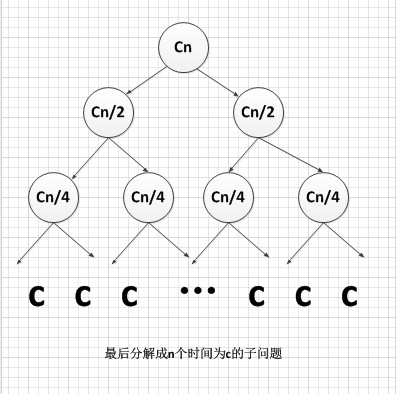https://blog.csdn.net/sinat_36306474/article/details/77906344

## 1.思路分析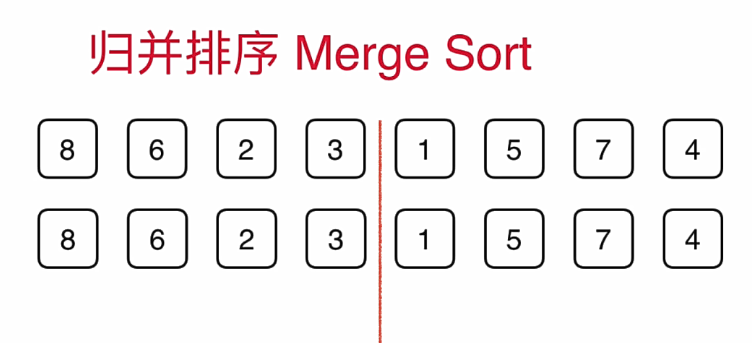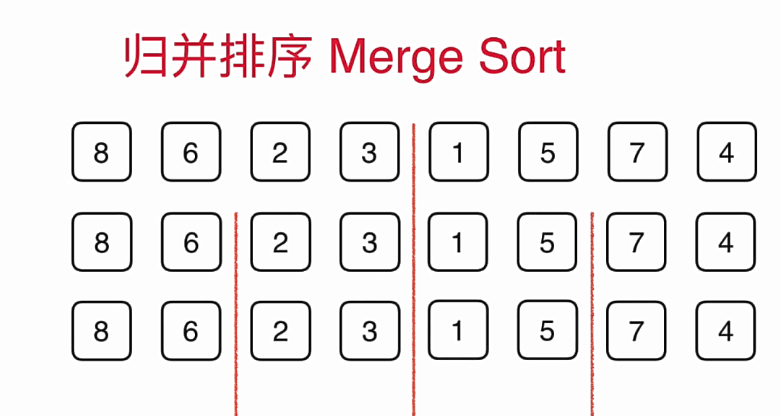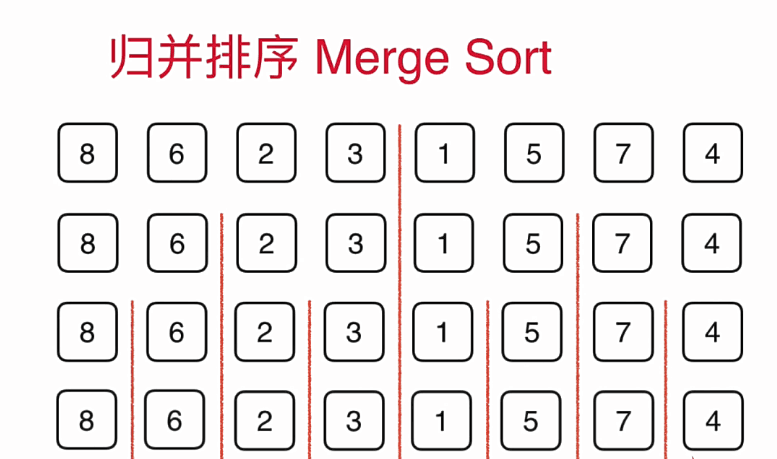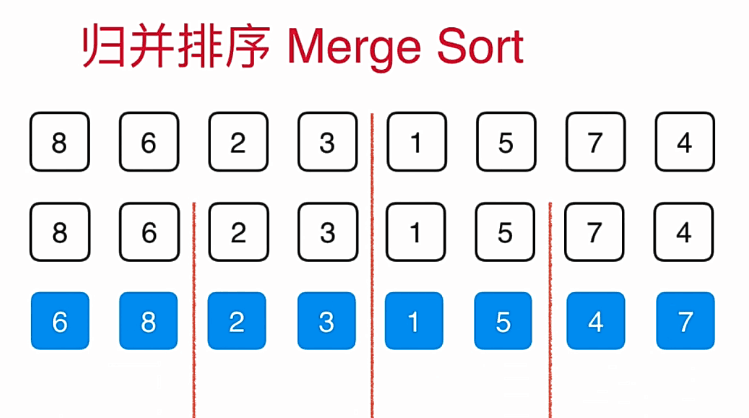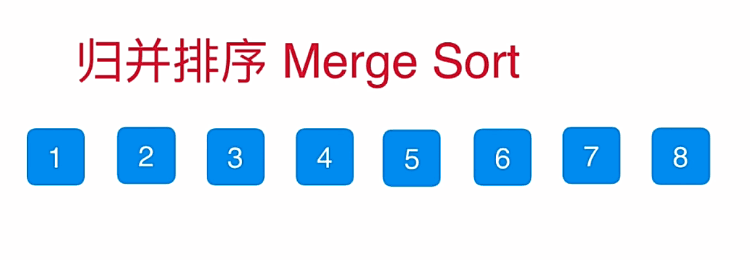*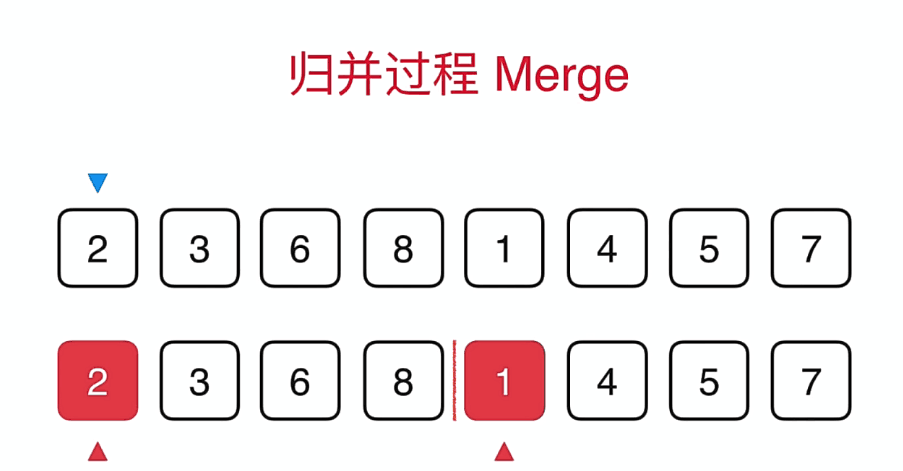*

*蓝色的箭头表示最终选择的位置，而红色的箭头表示两个数组当前要比较的元素，比如当前是2与1比较，1比2小，所以1放到蓝色的箭头中，**蓝色的箭头后移，1的箭头后移。***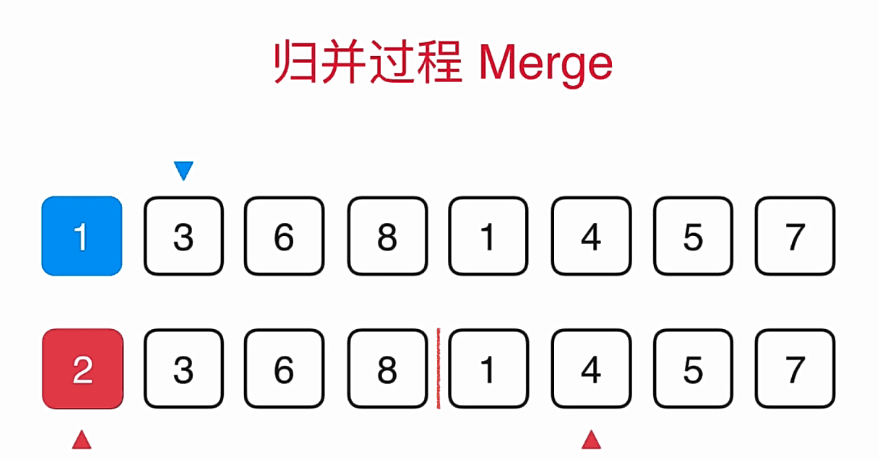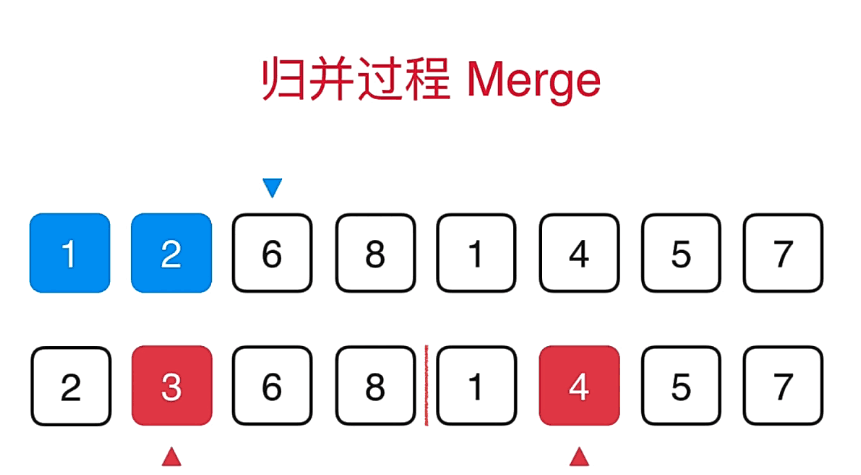## 2.代码实现

public class MergeSort {

public static void merge(int[] a, int low, int mid, int high) {
int[] temp = new int[high - low + 1];
int i = low;// 左指针
int j = mid + 1;// 右指针
int k = 0;
// 把较小的数先移到新数组中
while (i <= mid && j <= high) {
if (a[i] < a[j]) {
temp[k++] = a[i++];
} else {
temp[k++] = a[j++];
}
}
// 把左边剩余的数移入数组
while (i <= mid) {
temp[k++] = a[i++];
}
// 把右边边剩余的数移入数组
while (j <= high) {
temp[k++] = a[j++];
}
// 把新数组中的数覆盖nums数组
for (int k2 = 0; k2 < temp.length; k2++) {
a[k2 + low] = temp[k2];
}
}

public static void mergeSort(int[] a, int low, int high) {
int mid = (low + high) / 2;
if (low < high) {
// 左边
mergeSort(a, low, mid);
// 右边
mergeSort(a, mid + 1, high);
// 左右归并
merge(a, low, mid, high);
System.out.println(Arrays.toString(a));
}

}

public static void main(String[] args) {
int a[] = { 51, 46, 20, 18, 65, 97, 82, 30, 77, 50 };
mergeSort(a, 0, a.length - 1);
System.out.println("排序结果：" + Arrays.toString(a));
}
}


## 3.总结

O(n log n) 时间复杂度和常数级空间复杂度下，对链表进行排序。